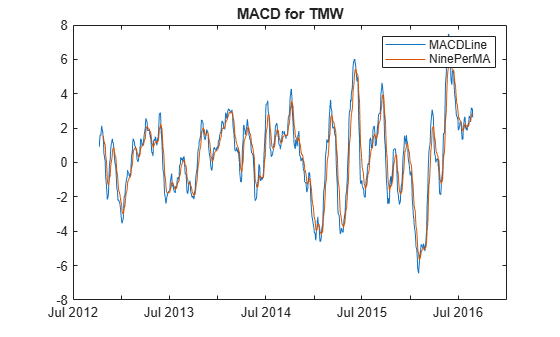# macd

Moving Average Convergence/Divergence (MACD)

Using a `fints` object for the `Data` argument of `macd` is not recommended. Use a matrix, `timetable`, or `table` instead for financial time series. For more information, see Convert Financial Time Series Objects fints to Timetables.

## Syntax

``[MACDLine,SignalLine] = macd(Data)``

## Description

example

````[MACDLine,SignalLine] = macd(Data)` calculates the Moving Average Convergence/Divergence (MACD) line from the series of data and the nine-period exponential moving average from the `MACDLine`.```

## Examples

collapse all

Load the file `SimulatedStock.mat`, which provides a timetable (`TMW`) for financial data for TMW stock.

```load SimulatedStock.mat [MACDLine, signalLine]= macd(TMW); plot(MACDLine.Time,MACDLine.Close,signalLine.Time,signalLine.Close); legend('MACDLine','NinePerMA') title('MACD for TMW')```## Input Arguments

collapse all

Data with high, low, open, close information, specified as a matrix, table, or timetable. For matrix input, `Data` is an `M`-by-`4` matrix of high, low, opening, and closing prices. Timetables and tables with `M` rows must contain variables named `'High'`, `'Low'`, `'Open'`, and `'Close'` (case insensitive).

Data Types: `double` | `table` | `timetable`

## Output Arguments

collapse all

MACD series, returned with the same number of rows (`M`) and type (matrix, table, or timetable) as the input `Data`. The `MACDLine` is calculated by subtracting the 26-period (7.5%) exponential.

Nine-period exponential series, returned with the same number of rows (`M`) and type (matrix, table, or timetable) as the input `Data`. The nine-period (20%) exponential moving average of the `MACDLine` is used as the `SignalLine`.